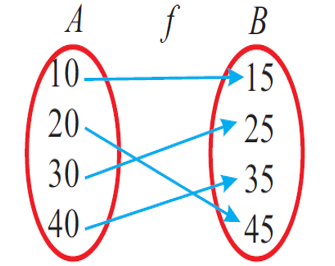Mathematics
How to Prove Bijection?
7.3k views

 1 Introduction 2 How to Tell if a Function is Invertible 3 Bijective Function examples 4 Summary 5 FAQs

12 October 2020

## Introduction

One to one function generally denotes the mapping of two sets. A function g is one-to-one if every element of the range of g matches exactly one element of the domain of g. Aside from the one-to-one function, there are other sets of functions that denotes the relation between sets, elements, or identities. They are;

• Many to One function
• Onto Function

### What is an invertible function?

In general, a function is invertible as long as each input features a unique output. That is, every output is paired with exactly one input. That way, when the mapping is reversed, it'll still be a function! Notice that the inverse is indeed a function.

## How to tell if a function is Invertible?

One major doubt comes over students of “how to tell if a function is invertible?”.

Let us define a function $$y = f(x): X → Y.$$ If we define a function g(y) such that $$x = g(y)$$ then g is said to be the inverse function of 'f'.

Think: If f is many-to-one, $$g: Y → X$$ won't satisfy the definition of a function.

So to get the inverse of a function, it must be one-one.

However if $$f: X → Y$$ is into then there might be a point in Y for which there is no x. This again violates the definition of the function for 'g' (In fact when f is one to one and onto then 'g' can be defined from range of f to domain of i.e. g: $$f(X) → X.$$

Hence, the inverse of a function might be defined within the same sets for X and Y only when it is one-one and onto.

Note: A monotonic function i.e. bijection function is usually invertible.

 Example

Let $$f : R → R$$ be defined as $$y = f(x) = x^2.$$ Is it invertible or not?

Solution:

No, it is not invertible as this is a many one into the function

This is many-one because for $$x = + a, y = a^2,$$ this is into as y does not take the negative real values.

 Example

Let f : R → [0, α) be defined as y = f(x) = x2. Is it invertible?

(see figure below)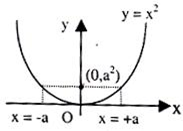Solution:

No, it is not an invertible function, it is because there are many one functions.

 Example

Let $$f : [0, α) → [0, α)$$be defined as $$y = f(x) = x^2.$$ Is it an invertible function? If so find its inverse.

Solution:

Yes, it is an invertible function because this is a bijection function. Its graph is shown in the figure given below.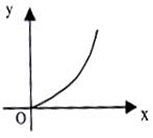Let y = x2 (say f(x))

$$\Rightarrow x = +\sqrt{y}$$

But x can be positive, as domain of f is [0, α)

$$\Rightarrow x = + \sqrt{y}$$

Therefore Inverse is $$y = \sqrt{x} = g(x)$$$$f(g(x)) = f(\sqrt{x}) = x, x> 0$$

$$g(f(x)) = g(x^2) = \sqrt{x^2} = x, x > 0$$

That is if f and g are invertible functions of each other then $$f(g(x)) = g(f(x)) = x$$

 Example

How are the graphs of function and the inverse function related? These graphs are mirror images of each other about the line y = x.

Solution:

Also, if the graph of $$y = f(x)$$ and $$y = f^{-1} (x),$$ they intersect at the point where y meets the line $$y = x.$$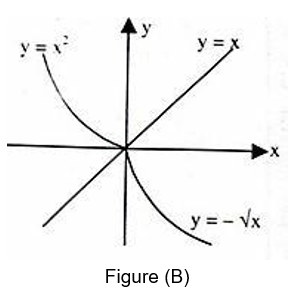Graphs of the function and its inverse are shown in figures above as Figure (A) and (B)

For Figure (A)

$y = f(x) = x^2; f : [0, ∞) → [0, ∞)$

## Bijective Function Examples

A function is called to be bijective or bijection, if a function f: A → B satisfies both the injective (one-to-one function) and surjective function (onto function) properties. It means that each and every element “b” in the codomain B, there is exactly one element “a” in the domain A so that f(a) = b. If the function proves this condition, then it is known as one-to-one correspondence. So let us closely see bijective function examples in detail.

### How to prove bijection?

Now, let us see how to prove bijection or how to tell if a function is bijective. If we want to find the bijections between two domains, first we need to define a map f: A → B, and then we can prove that f is a bijection by concluding that |A| = |B|. To prove f is a bijection, we must write down an inverse for the function f, or shows in two steps that

1. f is injective
2. f is surjective

If two sets A and B do not have the same elements, then there exists no bijection between them (i.e.), the function is not bijective. We think of a bijection as a “pairing up” of the elements of domain A with elements of codomain B. In fact, if |A| = |B| = n, then there exists n! bijections between A and B.

### Bijective Function Solved Problems

Let $$f : A \rightarrow B$$ be a function.

The function f is called as one to one and onto or a bijective function if f is both a one to one and also an onto function

More clearly,

f maps unique elements of A into unique images in B and every element in B is an image of element in A.

The figure shown below represents a one to one and onto or bijective function.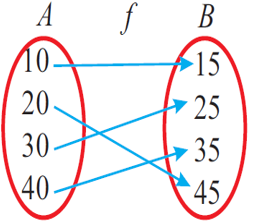Problem 1

Let $$f : A \rightarrow B. A, B$$ and $$f$$are defined as

A = {1, 2, 3, 4}

B = {5, 6, 7, 8}

f = {(1, 8), (2, 6), (3, 5), (4, 7)}

Verify whether f is a function. If so, what type of function is f ?

Solution :

Write the elements of f (ordered pairs) using an arrow diagram as shown below.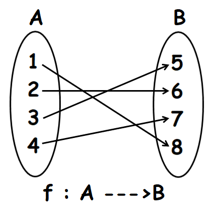In the above diagram, all the elements of A have images in B and every element of A has a distinct image.

That is, no element of A has more than one element.

So, f is a function.

Now every element of B has a preimage in A. So f is onto function.

Now every element of A has a different image in B.

That is, no two or more elements of A have the same image in B.

Therefore, f is one to one and onto or bijective function.

 Problem 2

Let $$f : X \rightarrow Y. X, Y$$ and $$f$$ are defined as

X = {a, b, c, d}

Y = {d, e, f}

$$f =$$ {(a, e), (b, f), (c, e), (d, d)}

Is $$f$$ bijective ? Explain.

Solution:

Write the elements of f (ordered pairs).

In the above equation, all the elements of X have images in Y and every element of X has a unique image.

That is, no element of X has more than one image.

So, f is a function.

Every element of Y has a preimage in X. So f is onto function.

The elements 'a' and 'c' in X have the same image 'e' in Y.

Because the elements 'a' and 'c' have the same image 'e', the above mapping can not be said as one to one mapping.

So, f is not bijective.

 Problem 3

Show that the function f(x) = 3x – 5 is a bijective function from R to R.

Solution:

Given Function: f(x) = 3x – 5

To prove: The function is bijective.

According to the definition of the bijection, the given function should be both injective and surjective.

## Summary

From the above examples we summarize here ways to prove a bijection

You have a function  $$f:A \rightarrow B$$ and want to prove it is a bijection. What can you do?

A bijection is defined as a function which is both one-to-one and onto. So prove that $$f$$ is one-to-one, and proves that it is onto.

### By size

If A and B are finite and have the same size, it’s enough to prove either that f is one-to-one, or that f is onto. A one-to-one function between two finite sets of the same size must also be onto, and vice versa. (Of course, if A and B don’t have the same size, then there can’t possibly be a bijection between them in the first place.)

Intuitively, this makes sense: on the one hand, in order for f to be onto, it “can’t afford” to send multiple elements of A to the same element of B, because then it won’t have enough to cover every element of B. So it must be one-to-one. Likewise, in order to be one-to-one, it can’t afford to miss any elements of B, because then the elements of have to “squeeze” into fewer elements of B, and some of them are bound to end up mapping to the same element of B. So it must be onto.

Note that we can even relax the condition on sizes a bit further: for example, it’s enough to prove that $$f$$ is one-to-one, and the finite size of A is greater than or equal to the finite size of B. The point is that f being a one-to-one function implies that the size of A is less than or equal to the size of B, so in fact, they have equal sizes.

### By inverse

One can also prove that $$f: A \rightarrow B$$ is a bijection by showing that it has an inverse: a function $$g:B \rightarrow A$$ such that $$g:(f(a))=a$$ and $$​​​​f(g(b))=b$$ for all $$a\epsilon A$$ and $$b \epsilon B$$, these facts imply that is one-to-one and onto, and hence a bijection. And it really is necessary to prove both $$g(f(a))=a$$ and $$f(g(b))=b$$ : if only one of these holds then g is called left or right inverse, respectively (more generally, a one-sided inverse), but f needs to have a full-fledged two-sided inverse in order to be a bijection.

One can also prove that $$f:A \rightarrow B$$ is a bijection by showing that it has an inverse: a function $$g:B \rightarrow A$$ such that  $$g(f(a))=a$$ and $$f(g(b))=b$$ for all $$a\epsilon A$$ and $$b \epsilon B$$, these facts imply $$f$$ that is one-to-one and onto, and hence a bijection. And it really is necessary to prove both $$g(f(a))=a$$ and $$f(g(b))=b$$: if only one of these holds then g is called left or right inverse, respectively (more generally, a one-sided inverse), but f needs to have a full-fledged two-sided inverse in order to be a bijection.

Written by Gargi Shrivastava

## What is Bijective function with an example?

In mathematics, a bijection, bijective function, one-to-one correspondence, or invertible function, maybe a function between two sets, where each element of a set is paired with exactly one element of the opposite set, and every element of the opposite set is paired with exactly one element of the primary set.

## Are all invertible functions Bijective?

Yes. A function is invertible if and as long as the function is bijective. ... A bijection f with domain X (indicated by $$f: X → Y$$ in functional notation) also defines a relation starting in Y and getting to X.

## What is the meaning of when a function is invertible?

In general, a function is invertible as long as each input features a unique output. That is, every output is paired with exactly one input. That way, when the mapping is reversed, it'll still be a function!. Notice that the inverse is indeed a function.

## Bijection proof?

Let $$f : A \rightarrow B$$ be a function.

The function f is called as one to one and onto or a bijective function if f is both a one to one and also an onto function

More clearly,

$$f$$ maps unique elements of A into unique images in B and every element in B is an image of element in A.

The figure shown below represents a one to one and onto or bijective function.